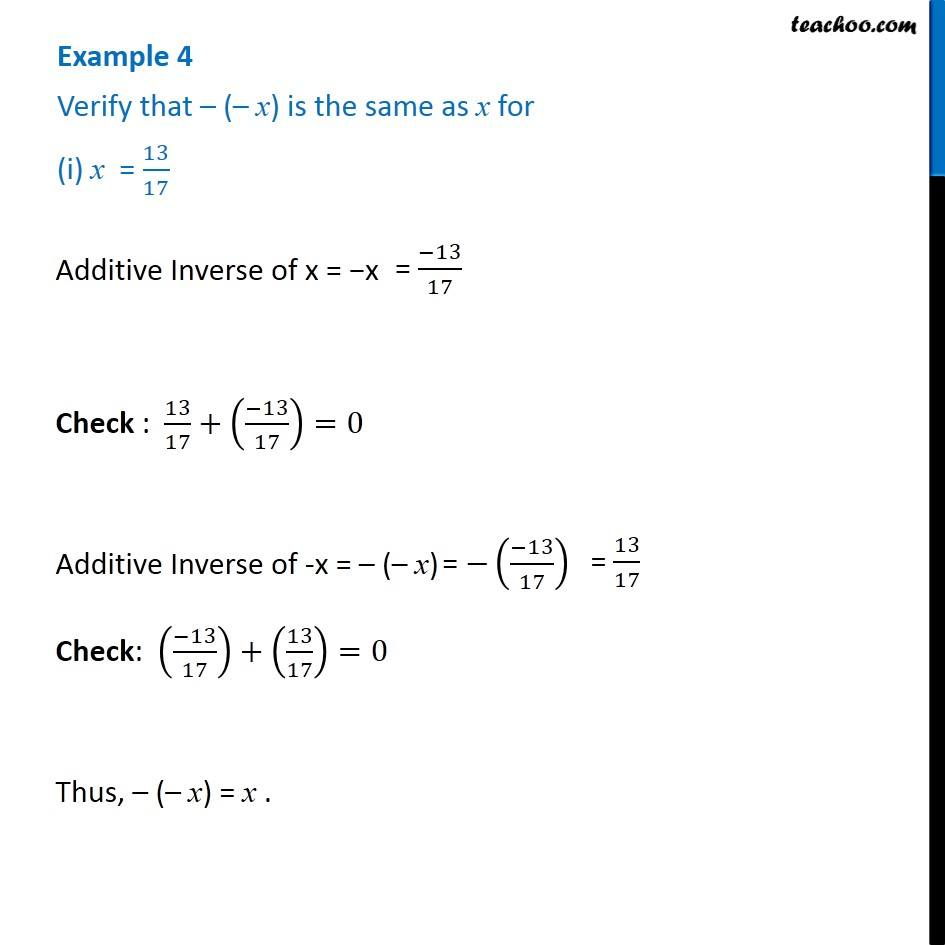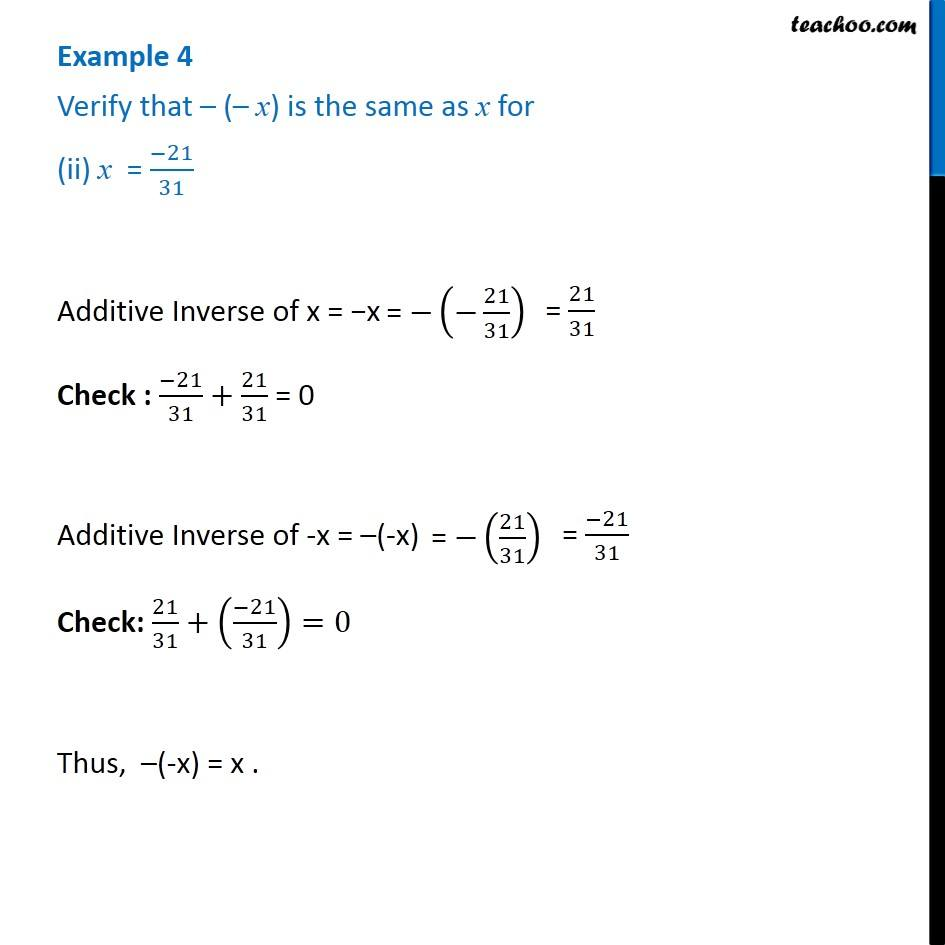Examples

Chapter 1 Class 8 Rational Numbers
Serial order wiseGet live Maths 1-on-1 Classs - Class 6 to 12

### Transcript

Example 4 Verify that – (– x) is the same as x for (i) x = 13/17 Additive Inverse of x = −x Check : 13/17+((−13)/17)=0 Additive Inverse of -x = – (– x) Check: ((−13)/17)+(13/17)=0 Thus, – (– x) = x . Example 4 Verify that – (– x) is the same as x for (ii) x = (−21)/31 Additive Inverse of x = −x Check : (−21)/31+21/31 = 0 Additive Inverse of -x = –(-x) Check: 21/31+((−21)/31)=0 Thus, –(-x) = x .